Student On-line Courses

# TI-Nspire™ CX II CAS - The Fundamentals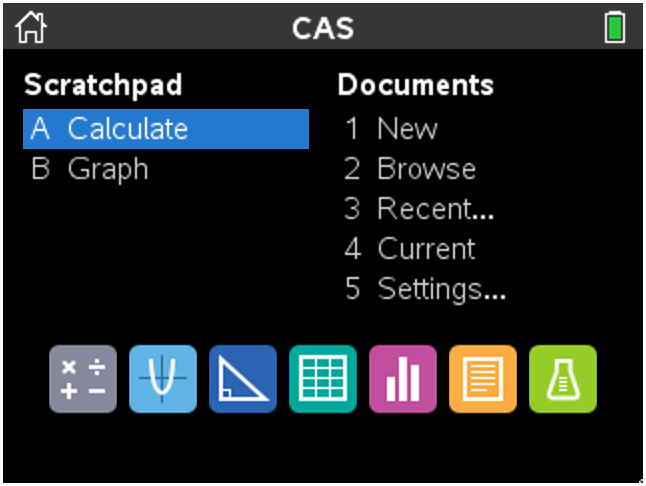To continue working on a document you already have open you would press: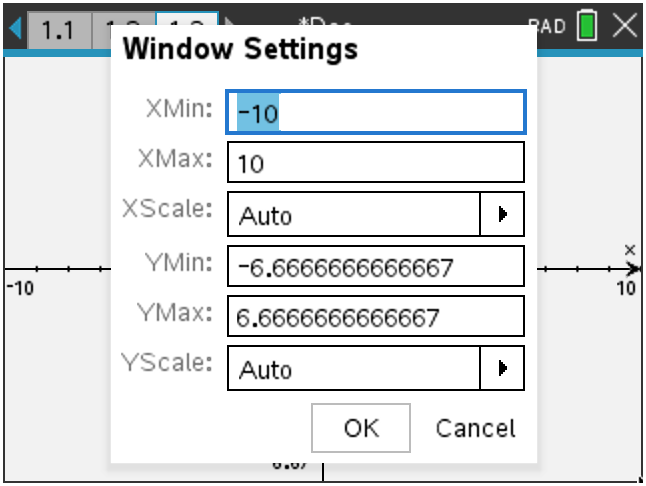To navigate from XMin to XMax press: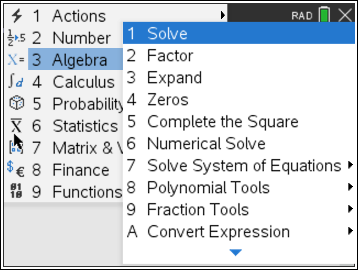Which of the following Algebra commands MUST include a comma followed by at least one pronumeral?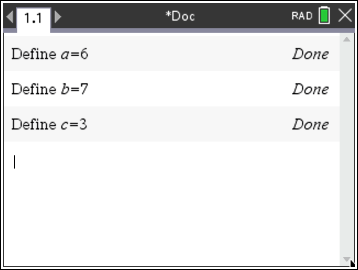To see all the defined variables you could press: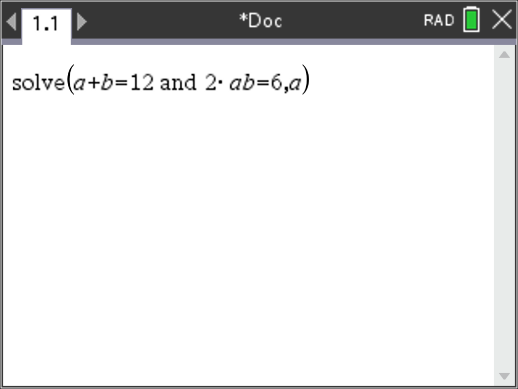The calculator will not solve these simultaneous equations because: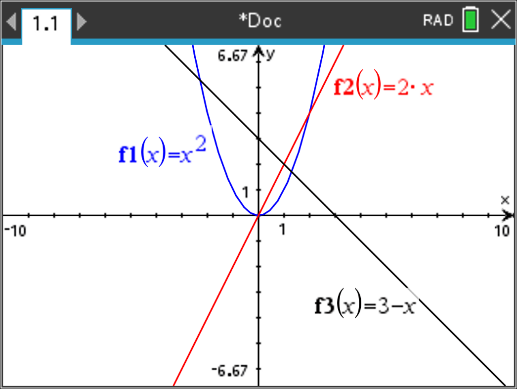Emily wants to generate a table of values for all three equations on a separate page, she could press: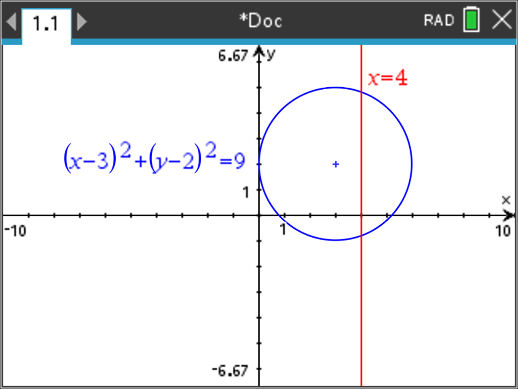A circle has been graphed using Relation 1. A vertical line has been graphed using Relation 2. Roger wants to find the y ordinate for the points of intersection using the solve command in the Calculator application. Roger could use: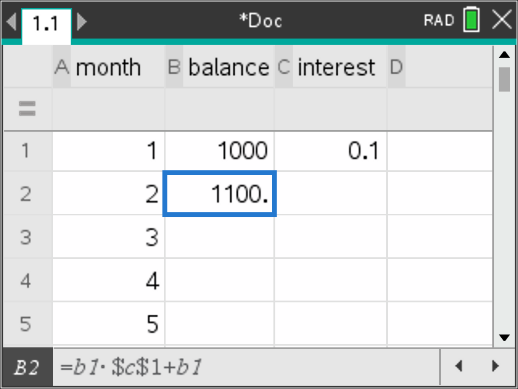The formula in cell B1 (shown at the bottom of the screen) is filled down to cell B5. The formula in cell B5 will be:

Page 1.2 identifies that this problem does not contain any lists, even though information is contained in the Spreadsheet application on page 1.1. This is because: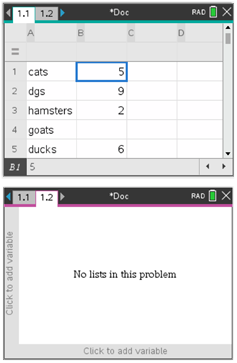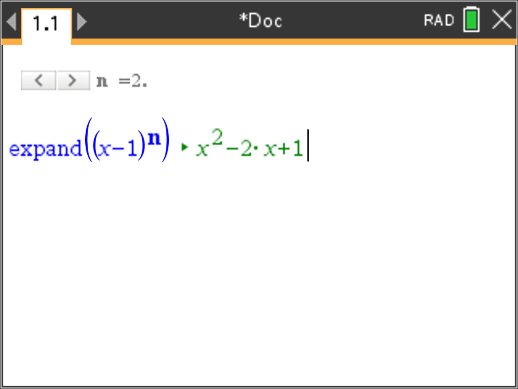Tim changes the value of the slider from n = 2 to n = 5. The coefficients in the second line will change from {1, -2, 1} to: